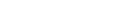# Variance Sum Law

## What is the Variance Sum Law?

The Variance Sum Law determines the variance of a sum (or difference) when you know the variance of the component parts.

For example, suppose you ran a research project which involved sampling the weight of apples in New York orchards, and then you did a similar project on oranges in Southern California. Now, imagine you need to work with the collected data from both research projects and draw some conclusions on the weight of apples and oranges. But first, what would the variance of your new data set be?

## The Variance Sum Law- Independent Case

If your two sets are independent, like the apples and oranges example, you can use the simplest version of the variance sum law.

Var(X ± Y) = Var(X) + Var(Y).

This just states that the combined variance (or the differences) is the sum of the individual variances.

This can also be written asSo if the variance of set 1 was 2, and the variance of set 2 was 5.6, the variance of the united set would be 2 + 5.6 = 7.6.

Need help with the formula? Check out our tutoring page!

## Sum and Difference

Note that the variance of the sum of both sets and the difference of both sets is exactly the same. This may take you by surprise on first sight, but after thinking it through you’ll realize that since Var(X-Y)=Var(X+(-Y))= Var(X) + Var(-Y) and Var(Y)= Var(-Y), Var(X+Y) must be equivalent to Var (X-Y).

## Variance Sum Law — Dependent Case

Now, what if your data sets are not independent? When you introduced dependence into your data sets the variance sum law becomes:

Since the population correlation coefficient ρ is defined as = Cov(x,y) / σxσy, this can also be expressed as

## References

Kotz, S.; et al., eds. (2006), Encyclopedia of Statistical Sciences, Wiley.
Vogt, W.P. (2005). Dictionary of Statistics & Methodology: A Nontechnical Guide for the Social Sciences. SAGE.

CITE THIS AS:
Stephanie Glen. "Variance Sum Law" From StatisticsHowTo.com: Elementary Statistics for the rest of us! https://www.statisticshowto.com/variance-sum-law/
---------------------------------------------------------------------------Need help with a homework or test question? With Chegg Study, you can get step-by-step solutions to your questions from an expert in the field. Your first 30 minutes with a Chegg tutor is free!